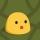Home
IT Knowledge
Inspiration
Languages
EN

# Python - min value in an array

0 points
Created by:Molly
201

This article will show you how to find the minimum value in an array in Python.

## Practical examples

### 1. With `min()` method

``````array = [3, 2, 7, 5]

print(min(array))  # 2``````

### 2. By comparing all the elements in the array

``````import math

def min_value(array):
result = math.inf

for item in array:
if item < result:
result = item

return result

numbers = [2, 3, 8, 1, 5]

print(min_value(numbers))  # 1``````